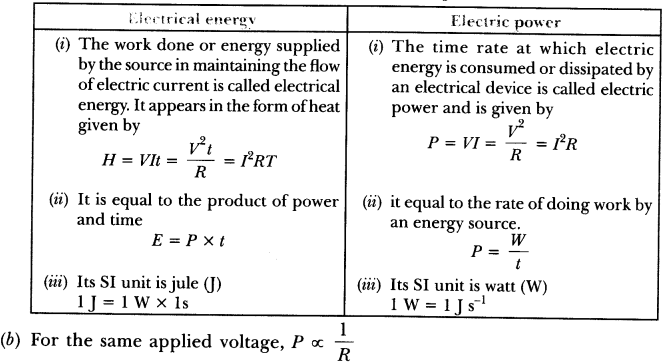# Some More Written Practice Questions

Question 1

(i) Draw a closed circuit diagram consisting of a 0.5 m long nichrome wire XY, an ammeter, a voltmeter, four cells of 1.5 V each and a plug key.
(ii)Following graph was plotted between V and I values :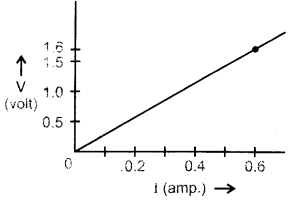What would be the values of V /I ratios when the potential difference is 0.8 V, 1.2 V and 1.6 V respectively? What conclusion do you draw from these values?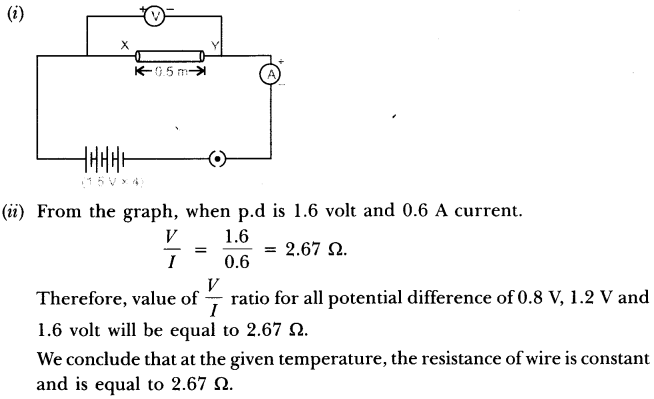Question 2

Study the following electric circuit and find (i) the current flowing in the circuit and (ii) the potential difference across 10 Ω resistor.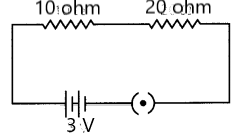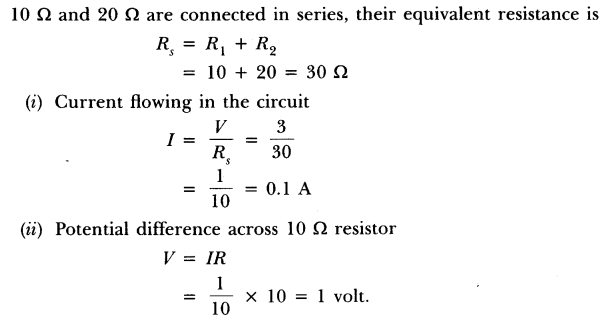Question 3.

Find the current drawn from the battery by the network of four resistors Shown in the figure.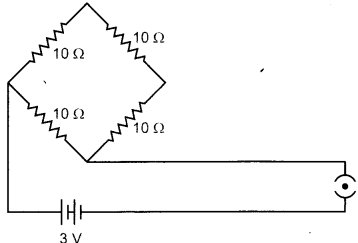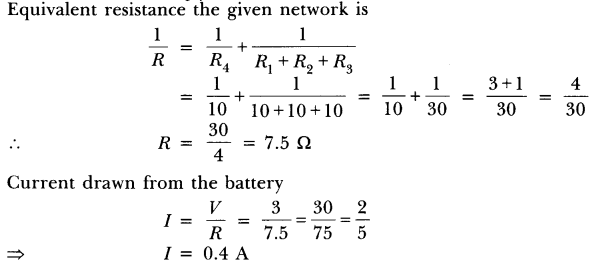Question.4

What is meant by electric current? Name and define its SI unit. In a conductor, electrons are flowing from B to A. What is the direction of conventional current? Give justification for your answer.
A steady current of 1 ampere flows through a conductor. Calculate the number of electrons that flows through any section of the conductor in 1 second. (Charge on electron 1.6 X 10-19 coulomb).

• Electric Current: The amount of charge ‘Q’ flowing through a particular area of cross section in unit time ‘t’ is called electric current, i.e.
• Electric current, I = Q/t
•  SI unit of electric current is ampere.
•  One ampere of current is that current which flow when one coulomb of electric charge flowing through a particular area of cross-section of the conductor in one second, i.e. 1A = 1 Cs-1.
•  The direction of conventional current is A to B, i.e. opposite to the direction of flow of electrons. In a metal, flow of electrons carrying negative charge constitutes the current. Direction of flow of electrons gives the direction of electronic current by convention, the direction of flow of positive charge is taken as the direction of conventional current.
• Charge = q = ne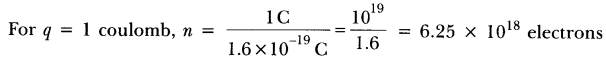Question.5

• Write two points of difference between electric energy and electric power.
• Out of 60 W and 40 W lamps, which one has higher electrical resistance when in use.
• What is the commercial unit of electric energy? Convert it into joules.